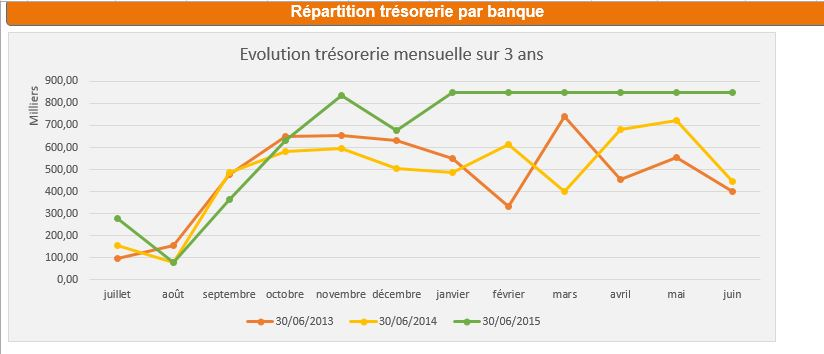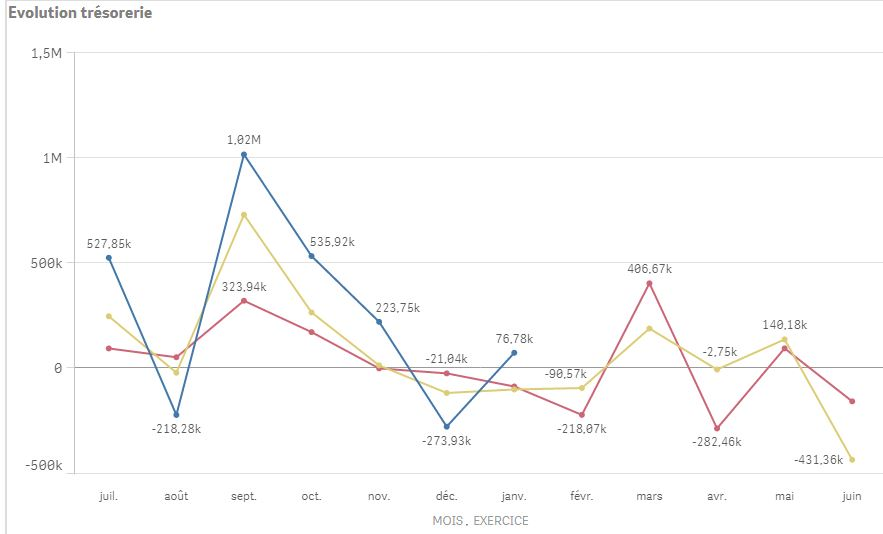# New to Qlik Sense

Discussion board where members can get started with Qlik Sense.

Announcements
Qlik® Product Spotlight: Discover what’s possible. Get more from our products.
See for yourself. Register today.Not applicable

## Graph in line - cumulated cash flow

Hi,

I would like to create a graph in line to analyse cumulated cashflow for a fiscal year with a comparison with precedent 2 years like this one.I have tried to create a mesure "TRESORERIE CUMULEE" with the expression (found in a forum) :

rangesum( above( sum({\$<FLAGTRESORERIE={1}>}SOLDE),0,rowno()))

But it doesn't work...I get this graph..My script :

SET DebutTreso1 = 50700000;
SET FinTreso1 = 50879999;
SET DebutTreso2 = 50900000;
SET FinTreso2 = 51779999;
SET DebutTreso3 = 51900000;
SET FinTreso3 = 51979999;
SET DebutTreso4 = 51990000;
SET FinTreso4 = 53999999;

...

[FEC RETRAITE]:
JournalCode as [CODE JOURNAL],
JournalLib as [LIBELLE JOURNAL],
EcritureNum as [NUMERO ECRITURE],
CompteNum as [NUMERO COMPTE],
CompteLib as [LIBELLE COMPTE],
CompAuxNum as [NUMERO COMPTE AUXILIAIRE],
CompAuxLib as [LIBELLE COMPTE AUXILIAIRE],
PieceRef as [REFERENCE PIECE],
PieceDate as [DATE PIECE],
EcritureLib as [LIBELLE ECRITURE],
If (Sens='C',Montant * -1,Montant) AS SOLDE,
Date(Date#([EcritureDate],'YYYYMMDD'),'DD/MM/YYYY') as [DATE ECRITURE],

If(CompteNum >=\$(DebutTreso1) and CompteNum <=\$(FinTreso1) or CompteNum >=\$(DebutTreso2) and CompteNum <=\$(FinTreso2) or CompteNum >=\$(DebutTreso3) and CompteNum <=\$(FinTreso3) or CompteNum >=\$(DebutTreso4) and CompteNum <=\$(FinTreso4),1,0) as FLAGTRESORERIE

FROM [lib://TDB MSB/FEC ORIGINAL.qvd] (qvd);

CALENDRIER:
*,
if(Num(MOIS)=7,1,
if(Num(MOIS)=8,2,
if(Num(MOIS)=9,3,
if(Num(MOIS)=10,4,
if(Num(MOIS)=11,5,
if(Num(MOIS)=12,6,
if(Num(MOIS)=1,7,
if(Num(MOIS)=2,8,
if(Num(MOIS)=3,9,
if(Num(MOIS)=4,10,
if(Num(MOIS)=5,11,
if(Num(MOIS)=6,12
)))))))))))) as FISCAL_MOIS_ID;

Date(Date#([EcritureDate],'YYYYMMDD'),'DD/MM/YYYY') as [DATE ECRITURE],
Date(Date#([ValidDate],'YYYYMMDD'),'DD/MM/YYYY') as [EXERCICE],
Date(Date#([EcritureDate],'YYYYMMDD'),'MM/YYYY') as [MOIS ANNEE],
Month(Date#([EcritureDate],'YYYYMMDD')) as MOIS,
Year(Date#([EcritureDate],'YYYYMMDD')) as ANNEE

FROM [lib://TDB MSB/FEC ORIGINAL.qvd] (qvd);

Thank you for your help

SincerelyNot applicable

## Re: Graph in line - cumulated cash flow

Hi everybody,

I'm sorry but I would like to raise the question again of the discussion that I put in this forum two days ago.

I need to create this chart but I don't succeed in finding the answer in internet. I need your helpI managed to create cumulative sum with qlikview by using "full accumulation" in the chart properties but it's quite difficult in qlik sense.

According to my research in this forum, I need to use Rangesum fonction but I don't understand how this fonction works.

Could you explain to me how I have tu use this fonction ? With RecNow ?

You ll find attached a example in excel of what i would like to do.

Thank you very much

Sincerely